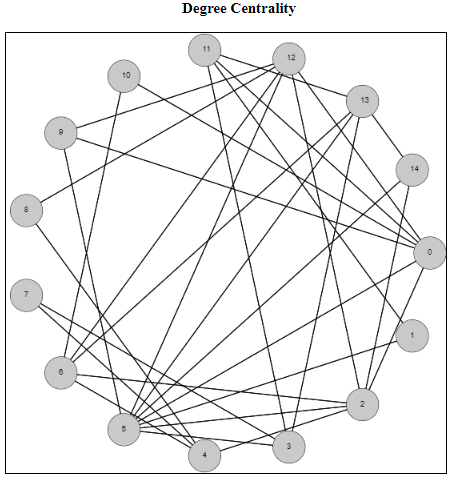# Degree Centrality of a GraphI wanted to spend some time on centrality measures of a graph. These are measurements of how important each node (or edge) is to the overall graph. But how do we define, or determine, importance? There is no unique way to answer this question, so there are varying metrics for measuring centrality. Which one you choose depends on several factors including how many other nodes of the graph are included, as well as the run time of the metrics you’re considering.

I have just published a script focusing on the degree centrality metric. The degree centrality metric is called a “walk metric” because it determines how important a node is by how many other nodes that can be reached by walks of up to a certain length. Lets look at the definition of the degree of a node to see if we can understand why it is called a walk metric.

In an undirected graph G = (V, E), the degree of a node u [in] V is the |{v | (u, v) [in] E}|. This is the size of the set of nodes that are connected to node u via a single edge. Another way of describing a single edge is a walk of length one. So the degree metric measures the importance of a node by the number of unique walks of length one.

The normalized degree centrality of a node v in a graph G = (V,E) measures how many nodes are connected to the node v, compared to the maximum possible number of edges that can be connected to this node. Because we are dealing with simple undirected graphs (at most a single edge between any two distinct vertices), this maximum possible number will always be |V – 1|. So the normalized degree can be calculated by dividing the degree of the node (the number of nodes it is connected to) by |V – 1|.

So for the example above, the node 0 has degree 6 because it is connected to nodes 2, 5, 9, 10, 11, and 12. There are 15 total nodes in this graph, so to calculate the normalized degree centrality of the node 0, it will be 6 / 14, which rounds to 0.428571.

To see more examples and to help answer questions, check out the script in my examples section on degree centrality